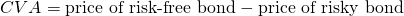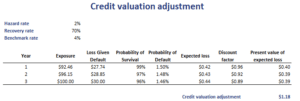What is CVA in finance? The Credit Valuation Adjustment (CVA) is a very important concept in fixed income. CVA is the sum of the present value of the expected loss for each period of a bond. In other words, CVA is the monetary value of the credit risk in present value terms. CVA is used by fixed income investors to assess the credit risk of bonds with default risk.

On this page, we discuss the credit valuation formula and discuss a numerical example of how investors typically calculate the CVA. The CVA calculator in Excel is available for download at the bottom of the page. In the spreadsheet, we calculate the CVA using the loss given default and the probability of default.

If we have both the price of the risky bond and an otherwise identical risk-free bond, then CVA can easily be calculated asIn practice, however, investors will not calculate the CVA like this. Instead, they will calculate the expected loss for each period using the probability of default and the loss given default. The following example in Excel illustrates how we would calculate the CVA in practice.

The best way to get the credit valuation adjustment explained, is using a numerical example using some real-life data. The table below illustrates how to calculate the credit valuation adjustment using an Excel spreadsheet. This template can easily be extended for bonds with a longer time to maturity. Note, however, that the example we consider below assumes we are dealing with a zero-coupon bond. Thus, we don’t need to consider coupon payments over the life of the bond.Summary

What is the credit valuation adjustment? We showed that CVA is the difference between the price of a risk free bond and an otherwise identical risky bond. It is used by fixed income investors to assess the riskiness of corporate bonds. The approach is easily implemented using a spreadsheet.

### CVA calculation

Want to have an implementation in Excel? Download the Excel file: CVA Calculator